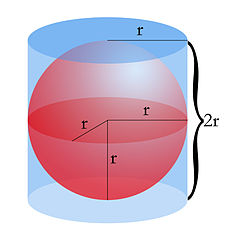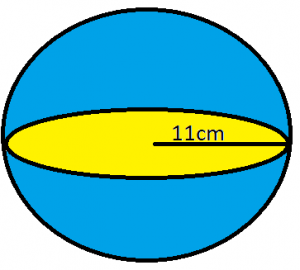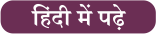# Volume of a Sphere: Definition, Formula and Examples

A sphere is a geometrical figure whose set of all points in three-dimensional space are at the same distance (the radius) from a given point (the centre), or the result of rotating a circle about one of its diameters. In order to determine the volume of a sphere, one must apply the formula required to calculate it. The sphere is also the one of the most important topics of geometry that is asked in the government recruitment exams. Generally, there are questions asked are related to basic concepts and formulas. To let you make the most of the Mathematics section

Here we are providing the definition, properties, formula with examples of a sphere to explain the students on the applications of the same.

### The volume of a sphere: Definition

sphere is a perfectly round geometrical object in 3-dimensional space. It can be characterized as the set of all points located equidistance (radius) away from a given point (center). It is perfectly symmetrical and has no edges or vertices.### Volume of a sphere: Formula

Greek philosopher Archimedes discovered The formula for the Volume of a sphere over two thousand years ago. He was the first person to state and prove that the volume of a sphere is exactly two thirds the volume of its circumscribed cylinder, which is the smallest cylinder that can contain the sphere. The spherical object is placed inside a solid container where the radius of the spherical object is equal to the radius of the circular bases of the cylinder. The Diameter of a sphere is equal to the height of the cylinder.Volume of a sphere= 2/3 volume of a Cylinder

Volume of a Sphere = 2/3 (πr2h); where r is the radius and h is the height of the cylinder

Now we know that height of cylinder= diameter of the sphere

Therefore,

Volume of a Sphere=  2/3 (πr2.2r); Diameter= 2x Radius

Volume of a Sphere= 4/3(πr3)

### Volume of a sphere: Example

Q. Radius of a sphere is 11cm. Calculate its volumeSolution: We are given the radius of the sphere= 11cm

We know that Volume of Sphere= 4/3(πr3)

Volume of sphere= 4/3 (3.14×113)

Volume of sphere= 5572.45 cm3

###You may also like to read:

×

Thank You, Your details have been submitted we will get back to you.Join India largest learning distination

What You Will get ?

•Job Alerts
•Daily Quizes
•Subject-Wise Quizes
•Current Affairs
•previous year question papers
•Doubt Solving session

ORJoin India largest learning distination

What You Will get ?

•Job Alerts
•Daily Quizes
•Subject-Wise Quizes
•Current Affairs
•previous year question papers
•Doubt Solving session

ORJoin India largest learning distination

What You Will get ?

•Job Alerts
•Daily Quizes
•Subject-Wise Quizes
•Current Affairs
•previous year question papers
•Doubt Solving session

Enter the email address associated with your account, and we'll email you an OTP to verify it's you.Join India largest learning distination

What You Will get ?

•Job Alerts
•Daily Quizes
•Subject-Wise Quizes
•Current Affairs
•previous year question papers
•Doubt Solving session

## Enter OTP

Please enter the OTP sent to
/6

Did not recive OTP?

Resend in 60sJoin India largest learning distination

What You Will get ?

•Job Alerts
•Daily Quizes
•Subject-Wise Quizes
•Current Affairs
•previous year question papers
•Doubt Solving sessionJoin India largest learning distination

What You Will get ?

•Job Alerts
•Daily Quizes
•Subject-Wise Quizes
•Current Affairs
•previous year question papers
•Doubt Solving session

## Almost there

+91Join India largest learning distination

What You Will get ?

•Job Alerts
•Daily Quizes
•Subject-Wise Quizes
•Current Affairs
•previous year question papers
•Doubt Solving session

## Enter OTP

Please enter the OTP sent to Edit Number

Did not recive OTP?

Resend 60

## By skipping this step you will not recieve any free content avalaible on adda247, also you will miss onto notification and job alerts

Are you sure you want to skip this step?

## By skipping this step you will not recieve any free content avalaible on adda247, also you will miss onto notification and job alerts

Are you sure you want to skip this step?